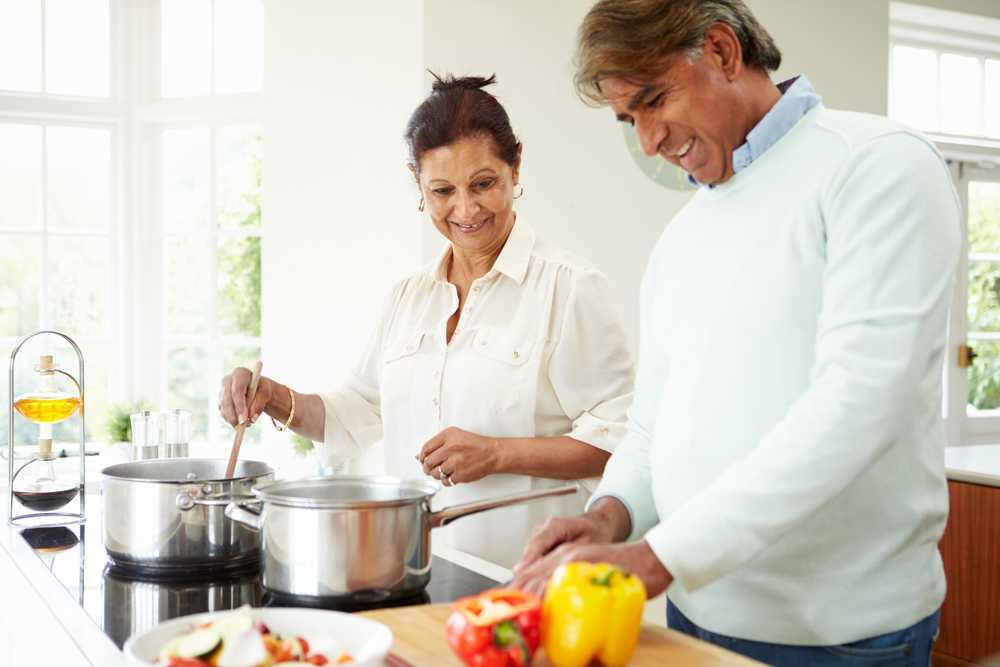# Calculating carb content of recipesYou can work out the carbohydrate content of meals and recipes by using the steps we’ve covered in the last few topics.

For working out the carbohydrate content in a recipe, follow the steps below:

• Identify each item from the ingredients list that contains carbohydrate
• Work out how much carbohydrate is in the portion of each ingredient that is to be counted
• Add together the carbohydrate value for each ingredient
• Divide the total carbohydrate by the number of each serving

## Worked example for calculating carbohydrate in recipes

Using the steps above, let’s calculate the carbohydrate content for the following recipe:

### Step 1: Identifying foods containing carbohydrate

In this meal, the items that we need to find out the carbohydrate content for are:

• Black beans
• White rice (cooked)
• Taco shells

### Step 2: Work out carbohydrate content of each item

Black beans

The total carbohydrate content for ½ a can is 17.7g. As we are using a full can, we need to double this figure, which equals 35.4g. We’ll round this down to 35g.

Taco shells

Each taco shell contains 7.7g of carbohydrate. As we need 8 in the recipe, we will multiply this by 8, which equals 61.6g. We’ll round this up to 62g.

White rice

From the Diabetes UK reference guide, we can get the values for cooked rice. 100g of cooked rice is 32g of carbohydrate. For the recipe, we need 150g so we need to work out what 50 grams are. As we have the value for 100 grams, we can get the value for 50 grams; we need to add this value to half of this value:

To do this we need to divide 32 by 2 which equals 16. If we add 32 and 16 together, we get the carbohydrate content for 150g of cooked rice = 48g.

So the carbohydrate content for all 3 items are:

The total carbohydrate content for the meal is 145g.

As the recipe serves 4 people, (and assuming that you are creating 4 equal sized portions), we would divide the total carbohydrate content by 4 which equals 36.25g. We’ll round this down to 36 grams per serving.

Now we know how to work out the carbohydrate content of foods and recipes, we’ll explain how you match this with your bolus insulin in the next few topics.